# Factorization Machines

Alex Egg,

To motivate the intuition for Factorization Machines, let’s consider a toy problem of trying to learn the XOR function.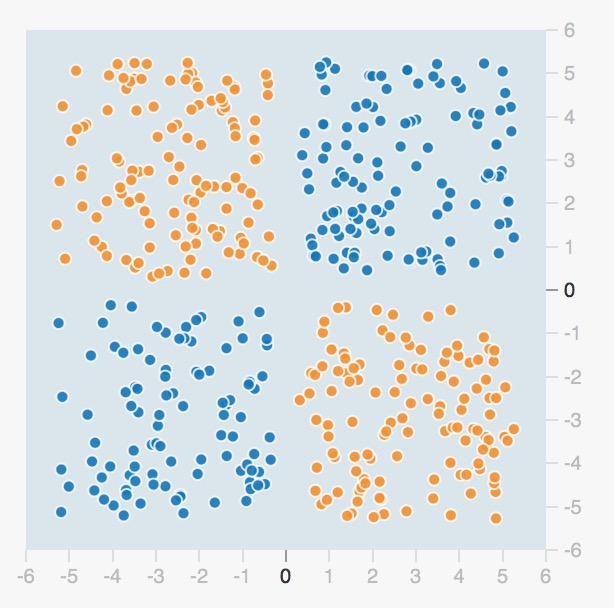A linear model cannot fit the data:

Where $n$ is the number of parameters in the dataset, which in this case is 2: x1 and x2.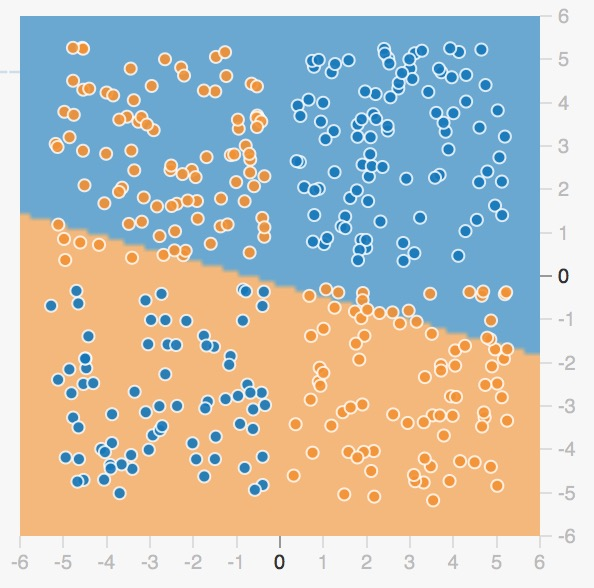However, a more sophisticated like a tree, Kernel-SVM or Neural Net model can (https://i.stack.imgur.com/v4IfD.png). Here’s a NN w/ 4 neurons in the hidden layer: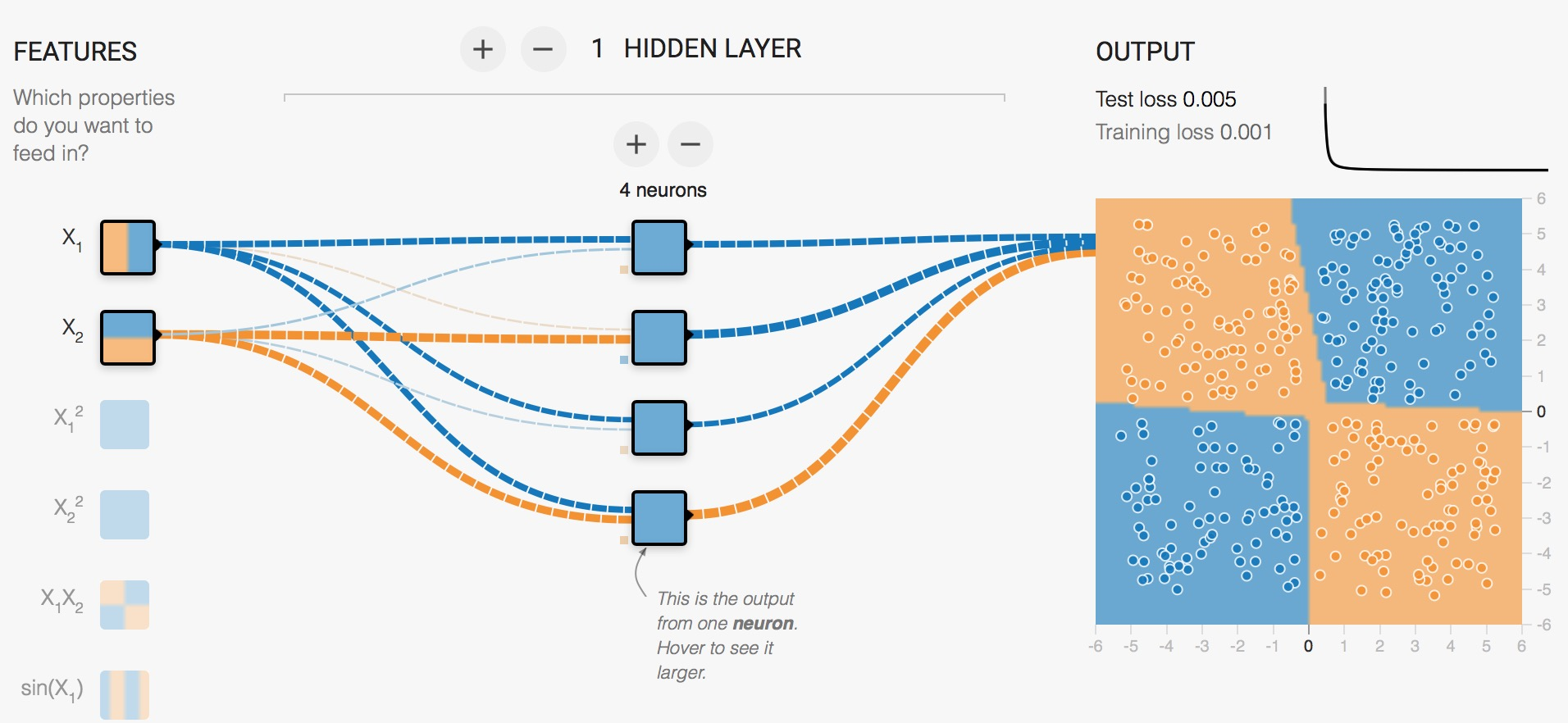However, if we do a sample feature cross with the only two features it becomes linearly separable in 3 Dimensions.

where $n$ is the number of features (dimensionality).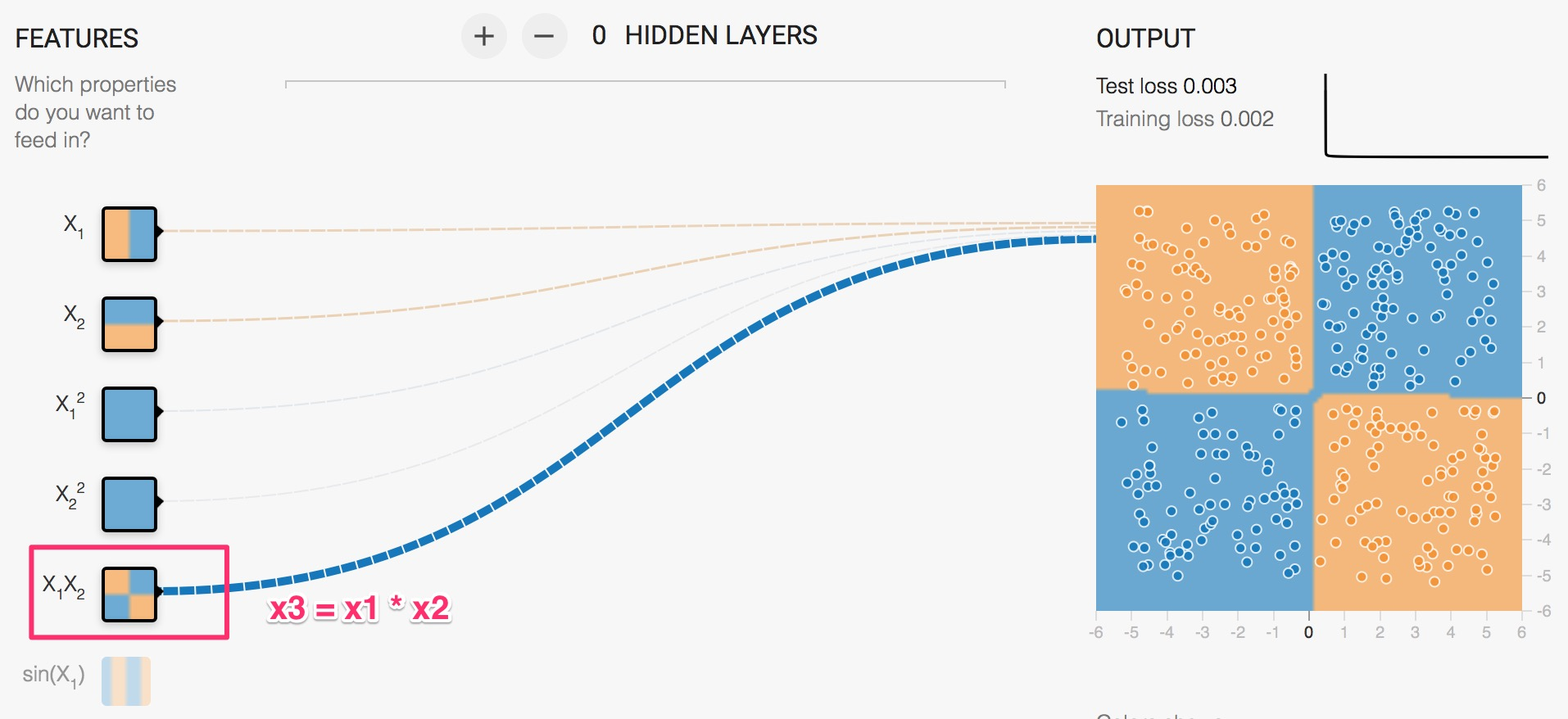As you can see the network ignores all other inputs and learns a near perfect fit using a linear model!

This is called Feature Crossing.

## Feature Crosses & Polynomial Regression

$x_3 = x_1 \times x_2$

Now in this 3-dimensional space, we can fit a plane through the two classes.

In other words doing creative feature engineering, we didn’t need to introduce a more complicated model like an non-linear SVM or Neural Net.

Now if we take this to it’s logical conclusion with an arbitrary number of features, then we would want to try all combinations of feature crosses. This called Polynomial regression, where you can imagine the feature space is insanely large. This also leads to overfitting due to the dimensionality growth.

In a simular vein to Feature Crosses, we add can exponentiate our input parameters to add more features:

$x_3 = x_2^2$

Now you have an $O(n^2)$ algorithm that learns $\binom{p}{2}$weights where $p$ is the number of features. As you can see the training time increases quadratically and so does the number of parameters.

## FMs

Let $w_{i,j}$ be the weight assigned to a feature pair. Let $w_{i,j} = <v_i, v_j>$ where $v_i$s are vectors in $\mathbb{R}^k$ .

So for each binary feature, learn an embedding vector.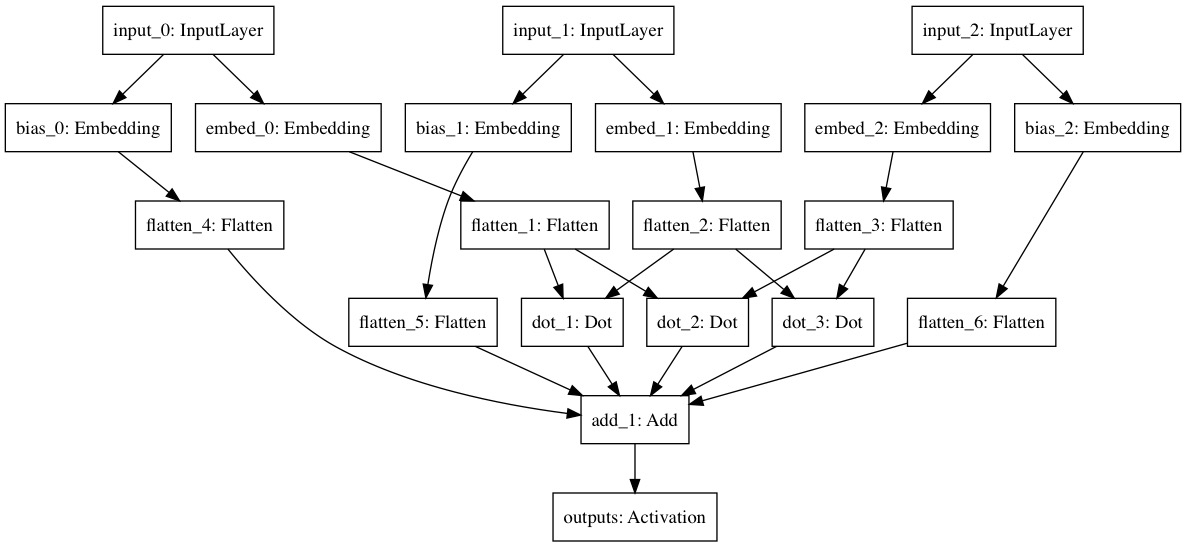Figure 1: FM for 3 features. Notice $\binom{3}{2}=3$ dot product interactions

Why does this run in linear time? I guess lema 3.1 in the FM paper shows how something can be pre-computed…

Image if we had 4 categorical features, each one-hot encoded, and it came out to 25 dimensions. That would come out to $\binom{25}{2}=300$ variables/weights to learn. Where if, instead I learned an embedding for each each feature, then I would only be learning $25\times k$ variables. If we choose $k=3$, then we only have to learn 75 parameters for roughly the same expressiveness as the 300 param model!

### Matrix Factorization

With only two features (users and items) we can recover classic matrix factorization.

User=Alice User=Bob User=Jane Movie=Titanic Movie=Avatar Rating?
1 0 0 0 1 1
0 1 0 1 0 0
0 0 1 1 0 1

This is the classic sparse User/Item matrix used in Matrix Factorization literature, where we learn 2 $k$ dimensional embeddings for user and items.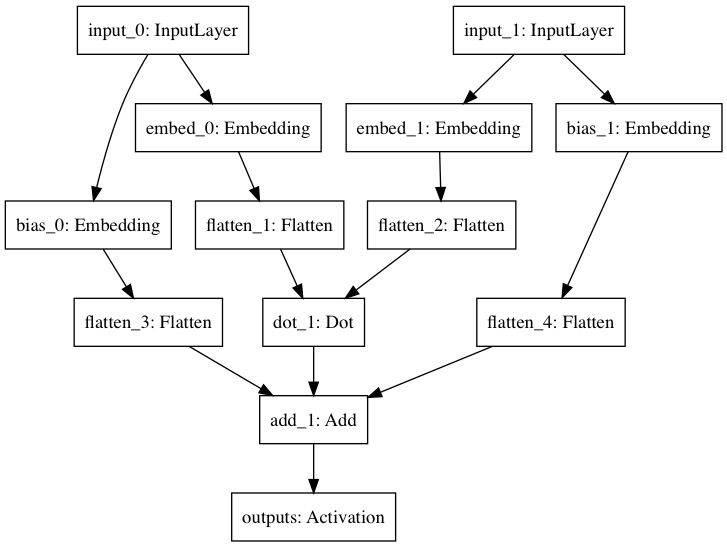Figure x: Graph export from Keras. The two embeddings are for users and items respectively. You can also see the bias embeddings too. The user/item interactions are modeled w/ the dot product and added to the biases for the final output. Where the two inputs are a user and item id.

MovieLens 10M contains roughly 71k users and 10k movies. If we made this into a sparse one-hot representation, it would be 81k dimensions and require $\binom{81000}{2}=3e10$, that’s 3 billion parameters (or 24 gigabytes of 64 bit floats)! But instead using smart feature crosses of learn embeddings we can learn it with only $81,000 \times k$ parameters.